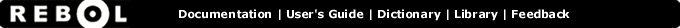# And - Function Summary

## Summary:

Returns the first value ANDed with the second.

## Usage:

and value1 value2

## Arguments:

value1 - The value1 argument. (must be: logic number char tuple binary image)

value2 - The value2 argument. (must be: logic number char tuple binary image)

## Description:

For LOGIC values, both values must be TRUE to return TRUE, otherwise a FALSE is returned. For integers, each bit is separately affected (a numerical AND). All arguments are fully evaluated.

 ``` print true and true true```

 ``` print true and false false```

 ``` print (10 < 20) and (20 > 15) true```

 ``` print 123 and 1 1```

 ``` print 1.2.3.4 and 255.0.255.0 1.0.3.0```

## Related:

all - Shortcut AND. Evaluates and returns at the first FALSE or NONE.
integer? - Returns TRUE for integer values.
logic? - Returns TRUE for logic values.
not - Returns the logic complement.
or - Returns the first value ORed with the second.
xor - Returns the first value exclusive ORed with the second.